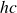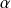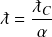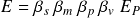# Rydberg constant

The Rydberg constant is a number for calculating the ground state energy of an electron. The constant is part of the Rydberg energy formula which multiplies the Rydberg constant by the energy constantto produce the correct ground state energy.

The elementary form of the Rydberg constant contains four operators but no maximum potentials

The Planck length is normally a maximum potential in historical constants, but in this case the Planck length is only used to remove another unnecessary quantity of Planck length in the energy constant.

The Rydberg constant includes all four operators in the expanded form of the energy formula. The spin operator is present in the ratio one-half; the rest mass operator appears in the ratio of the electron’s rest mass to the Planck mass; and for the ground state of an electron, the momentum and velocity operators are equal to, the fine-structure constant. This is evident in the wavelength and velocity of the ground state electron.

Given the electron’s rest mass, its wavelength in the ground state isand its velocity is.

The following equivalent form of the Rydberg constant replaces the fine-structure constant with dimensionless ratios of wavelength and velocity in the ground state electron

The four operators appear in the New Foundations Model energy formula–proportionally transforming the maximum Planck energy potentialThe natural formula expressing wavelength in terms of rest mass is

Rydberg constant